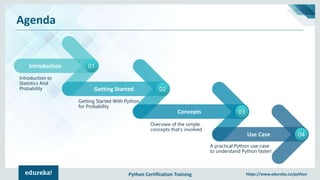Successfully reported this slideshow.

# Statistics Using Python | Statistics Python Tutorial | Python Certification Training | EdurekaYouTube videos are no longer supported on SlideShare×

# Statistics Using Python | Statistics Python Tutorial | Python Certification Training | Edureka

** Python Certification Training: https://www.edureka.co/python **
This Edureka PPT on Python Tutorial covers all the basic knowledge of statistics and probability for Python.

Why Python for Statistics?
What is Probability?
Data and Distribution
Revisiting the Normal
Poker Prediction Use-Case

Check out our Python Tutorial Playlist: https://goo.gl/WsBpKe
Follow us to never miss an update in the future.

Instagram: https://www.instagram.com/edureka_learning/

** Python Certification Training: https://www.edureka.co/python **
This Edureka PPT on Python Tutorial covers all the basic knowledge of statistics and probability for Python.

Why Python for Statistics?
What is Probability?
Data and Distribution
Revisiting the Normal
Poker Prediction Use-Case

Check out our Python Tutorial Playlist: https://goo.gl/WsBpKe
Follow us to never miss an update in the future.

Instagram: https://www.instagram.com/edureka_learning/

### Statistics Using Python | Statistics Python Tutorial | Python Certification Training | Edureka

1. 1. Python Certification Training https://www.edureka.co/python Agenda
2. 2. Python Certification Training https://www.edureka.co/python Agenda Introduction 01 Introduction to Statistics And Probability Getting Started 02 Concepts 03 Use Case 04 Getting Started With Python for Probability A practical Python use-case to understand Python faster! Overview of the simple concepts that’s involved
3. 3. Python Certification Training https://www.edureka.co/python Why Python For Statistics?
4. 4. Python Certification Training https://www.edureka.co/python Why Python For Statistics? R is a language dedicated for statistics! Then why Python? Building complex analysis pipelines that mix statistics with Image Analysis, Text Mining etc.. Here, the richness of Python is an invaluable asset!
5. 5. Python Certification Training https://www.edureka.co/python What is Probability?
6. 6. Python Certification Training https://www.edureka.co/python What is Probability? What is the chance of an event happening? How do you answer this? We need to consider all the other events that can occur before coming to a conclusion!
7. 7. Python Certification Training https://www.edureka.co/python The Coin Toss What are the outcomes for a coin toss? Flipping a heads Flipping a tails Any other outcome? NO! We call this the Sample Space!
8. 8. Python Certification Training https://www.edureka.co/python The Coin Toss What are the outcomes for a coin toss? A 100 Heads and 10 Tails, is this fair? Yes, the outcome here is to gather data, use statistics to make predictions and compare!
9. 9. Python Certification Training https://www.edureka.co/python The Coin Toss – Data Generation
10. 10. Python Certification Training https://www.edureka.co/python Too early for code?
11. 11. Python Certification Training https://www.edureka.co/python The Coin Toss – Code import random def coin_trial(): heads = 0 for i in range(100): if random.random() <= 0.5: heads +=1 return heads def simulate(n): trials = [] for i in range(n): trials.append(coin_trial()) return(sum(trials)/n) simulate(10) >> 5.4 simulate(100) >>> 4.83 simulate(1000) >>> 5.055 simulate(1000000) >>> 4.999781
12. 12. Python Certification Training https://www.edureka.co/python The Coin Toss – The Theory Given enough data, statistics enables us to calculate probabilities using real-world observations
13. 13. Python Certification Training https://www.edureka.co/python The Coin Toss – Python What are the chances of someone developing a disease over time? What is probability that a critical car component will fail when you are driving? Python making our lives simpler with this!
14. 14. Python Certification Training https://www.edureka.co/python Data And Distribution
15. 15. Python Certification Training https://www.edureka.co/python Data And Distribution Let’s tackle “Which wine is better than average” You need to know the nature of the data! Normal Distribution Normal distribution refers to a particularly important phenomenon in the realm of probability and statistics.
16. 16. Python Certification Training https://www.edureka.co/python Data And Distribution The high point in a normal distribution represents the event with the highest probability of occurring!
17. 17. Python Certification Training https://www.edureka.co/python Revisiting The Normal
18. 18. Python Certification Training https://www.edureka.co/python Revisiting The Normal Two major factors Central Limit Theorem Three Sigma Rule Central Limit Theorem dictates that the distribution of the estimates will look like a normal distribution. The Three Sigma rule dictates that given a normal distribution, 68% of your observations will fall between one standard deviation of the mean. 95% will fall within two, and 99.7% will fall within three. Learning Python
19. 19. Python Certification Training https://www.edureka.co/python Z-Score Learning Python
20. 20. Python Certification Training https://www.edureka.co/python Use-Case: Poker Probability
21. 21. Python Certification Training https://www.edureka.co/python Use-Case: Poker Prediction Can we predict the outcome of probability of occurrence of a poker hand?
22. 22. Python Certification Training https://www.edureka.co/python Use-Case: Poker Prediction Let’s look at the basics 52 cards in a standard deck! 4 of each shape For an Ace - P(A) = 4/52
23. 23. Python Certification Training https://www.edureka.co/python Use-Case: Poker Prediction Poker Without Python Poker With Python Texas Hold’em Pre-Flop: Each player is dealt two cards, known as "hole cards" Flop: Three community cards are dealt Turn: One community card is dealt River: Final community card is dealt
24. 24. Python Certification Training https://www.edureka.co/python Use-Case: Poker Prediction Dependent Events: Flush Draw Your Hand Community Cards
25. 25. Python Certification Training https://www.edureka.co/python Use-Case: Poker Prediction Dependent Events: Open-Ended Straight Draw Your Hand Community Cards
26. 26. Python Certification Training https://www.edureka.co/python Use-Case: Poker Prediction Involve Opponents now! Your Hand Community Cards Opponent’s Hand Total Pot = \$60 Opponents Bet = \$20
27. 27. Python Certification Training https://www.edureka.co/python Conclusion
28. 28. Python Certification Training https://www.edureka.co/python Conclusion